# Excel - Did You Know !

I Love Challenges in MS-Excel
Where there is a will there are many ways. Finding one that works for you is the challenge!
Published:
Updated:
Excel can be a tricky bit of software to get your head around. Whilst you’ll be able to eventually get to grips with the basic understanding of how to get by, there are a few Excel tips that not everybody will even know about let alone know how to do.

The latest info graphic lets take a look that are pretty lesser-known to most:

1. So to start with we often save Excel file and we forgets the path of the file we are working. There is very simple Excel Function here, which is called "Cell". Type in any blank cell of that workbook =Cell("filename"), the movement you press enter, it will show you the path of the file you are working on. Pretty simple & short.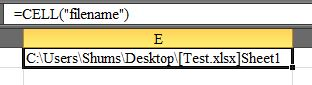2. Often typing a Function or a Formula, if you want to see the rest of the argument of that Function, start with any Function, for example if I type =vlookup and I want to see rest of the argument, =vlookup(Ctrl+Shift+A), arguments will appear in front of you like this =VLOOKUP(lookup_value,table_array,col_index_num,range_lookup), so you can see what would be complex formula and helps you making the combination, right in front of you.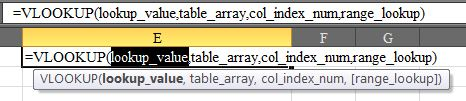3. Often typing Formulas or Function, we get mixed up and Errors and we don't know what to do. So let Excel to help us in Formula Auditing. For example, you have 1 in Cell A1, 2 in Cell A2 & abc in Cell A3 and I put formula =Sum(A1:A3)*A3, which will end up with #Value!, now you want to check, why there was error, then navigate to Formula/Evaluate Formula or press the yellow error sign on lower right of the cell and press Show Calculation Step, on the pop window you will see your formula, press Evaluate, it will show you which argument is giving you error, like in my formula its shows error *A3, then press Step In, it will show you why it is error, in my example it says "The cell currently being evaluated contains a constant."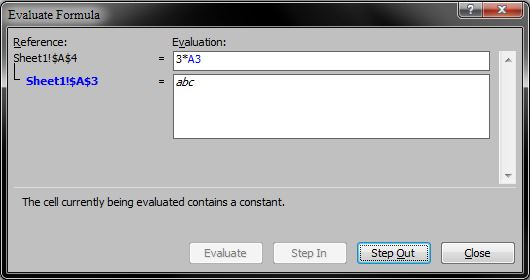By this you can see, which part of your formula is functioning and which one is not working.

4. Its often very inconvenient, if you have to go very end of the column of the sheet and see what is in that last column, when you have a vast number of columns. Lets make it easy. For example, I have multiple numbers in one row to few columns, simply type lookup formula with any scientific number, such as =lookup(1E100,1:1) select the whole row of that column and press enter, it will show you the cell value of the very end of the column.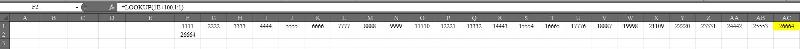Even if you change any number after last column, it will change the last cell value automatically.

5.If you ever come across the spreadsheet with lots of formulas spread across your data? This is one of the hidden function which would make it easy for you to see what is the Formula or Function behind the specific number. For example if I have 11 in E1, 22 in E2, 33 in E3 and in E4 I have formula =Sum(E1:E3), it give me number 66. You can see the formula in Formula Bar, suppose someone wants to hide the formula and sends you any data file, where you cannot see the formula bar like below image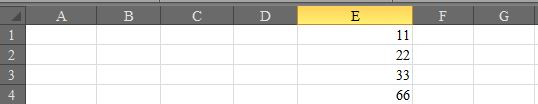For such instances, there is key called "Back Tricky" or "Caret Key" on top of your keyboard after Esc button.

Press Ctrl + ¬ and you will see the formula like below: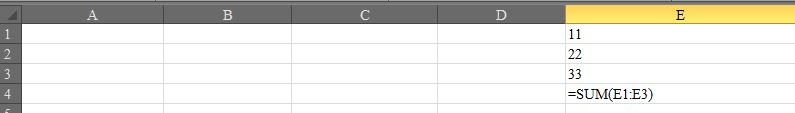If you press again, it will go back to normal window, where you cannot see the Formula.

6. Most of us knows how to get current date or time in cell by different formulas, but very few knows how to get current date or time in a cell with Keyboards.

• To enter Current Date in a cell Press Ctrl+; (semicolon ) it will inserts Current Date in your current Cell.
• To enter Current Time  in a cell Press Ctrl+Shift+: (colon ) it will inserts Current Time in your current Cell.

This works in almost all the Excel versions.

This is my first article, if you already knew some of the above stuff, then please appreciate my initiative.

28
2,108 Views
I Love Challenges in MS-Excel
Where there is a will there are many ways. Finding one that works for you is the challenge!

Commented:
nice ideas!

Commented: